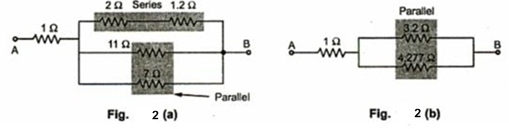### Summary of Series and Parallel circuits

The table 1 gives the equivalent at 'n' basic elements in series.Table . 1  Series combination of elements

The table 2 gives the equivalent at 'n' basic elements in parallel.Table . 2  Parallel combination of elements

Key point : The current through series combination remains same and voltage gets divided while in parallel combination voltage across combination remains same and currents gets divided.

Example 1 :
Find the equivalent resistance between the tow point A and B shown in the Fig. 1.Fig. 1

Solution : Identify combinations of series and parallel resistances.
The resistance 5 Ω and 6 Ω are in series, as going to carry same current.
So equivalent resistance is 5+6 = 11 Ω
While the resistance 3 Ω, 4 Ω and 4 Ω are in parallel, as voltage across them same but current divides.
...    equivalent resistance is,                1/R = 1/3 + 1/4 + 1/4 =10/12
...                                                         R = 12/10 = 1.2 Ω
Replacing these combinations redraw the figure as shown in the Fig. 2(a).
Now again 1.2 Ω and 2 Ω are in series so equivalent resistance is 2 + 1.2 = 3.2 Ω while 11 Ω and 7 Ω are in parallel.
Using formula (R R2 )/( R1 + R2 ) equivalent resistance is (11x 7)/(11+7) = 77/18 = 4.277 Ω
Replacing the respective combinations redraw the circuit as shown in the Fig. 2(b).
Now 3.2 and 4.277 are in parallel.
Replacing them by (3.2 x 4.227)/(3.2 + 4.227 ) = 1.8304 Ω
RAB  = 1+1.8304 = 2.8304 ΩFig. 2